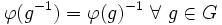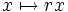# Isomorphism of groups

VIEW RELATED: Analogues of this | Variations of this | Opposites of this |[SHOW MORE]

## Definition

### Definition from first principles

Suppose$G$ and$H$ are groups. A function$\varphi:G \to H$ is termed an isomorphism of groups if$\varphi$ is bijective (i.e., it is both injective and surjective) and satisfies the following three conditions:

1.$\varphi(e) = e$
2.$\varphi(g^{-1}) = \varphi(g)^{-1} \ \forall \ g \in G$
3.$\varphi(gh) = \varphi(g)\varphi(h) \ \forall \ g,h \in G$

(It turns out that condition (3) guarantees the other two conditions).

If an isomorphism exists between two groups, we say that the group are isomorphic.

Note that there may exist more than one isomorphism between two groups. Specifying an isomorphism between groups is thus more than just saying that the groups are isomorphic.

### Definition in terms of concept of homomorphism

Suppose$G$ and$H$ are groups. A function$\varphi:G \to H$ is termed an isomorphism of groups if it satisfies the following equivalent conditions:

1.$\varphi$ is injective, surjective and is a homomorphism of groups
2.$\varphi$ is a homomorphism of groups, and it has a two-sided inverse$\varphi^{-1}:H \to G$ that is also a homomorphism of groups

### Definition in terms of changing the labels on the multiplication table

An isomorphism of groups$G$ and$H$ gives a rule to change the labels on the elements of$G$, so as to transform the multiplication table of$G$ to the multiplication table of$H$. (this description makes most sense when we're looking at finite groups).

### Equivalence of definitions

Further information: Equivalence of definitions of isomorphism

## Examples

### Extreme examples

1. Any two groups of order one are isomorphic, where the isomorphism sends the unique element of one group to the unique element of the other. For practical purposes, we think of there being only one group of order one, that we called the trivial group.
2. The identity map from a group to itself is an isomorphism.

### Examples in Abelian groups

Here are examples relating two additive structures:

1. The map$x \mapsto 2x$ gives an isomorphism between the additive group of all integers, and the additive group of all even integers.
2. The map$x \mapsto ix$ gives an isomorphism between the additive group of real numbers, and the additive group of purely imaginary numbers.
3. The map$x \mapsto rx$, for any nonzero rational number, gives an isomorphism from the additive group of rational numbers, to itself. (Note: This is an example of an isomorphism from a group to itself, other than the identity map.)

Here are examples relating an ostensibly additive structure with an ostensibly multiplicative structure, via :

1. The map$x \mapsto e^x$ gives an isomorphism between the additive group of all real numbers$(\R,+)$, and the multiplicative group of positive real numbers$(\R^{*+},\times)$. The inverse to this isomorphism is the logarithm map.

### Examples in Abelian groups involving modular arithmetic

1. Consider the additive group of integers mod 2, and the multiplicative group of nonzero integers mod 3. The map$x \mapsto 2^x$ gives an isomorphism of these groups.
2. Consider the group of integers mod$n$, and the group of$n^{th}$ roots of unity. If$\zeta$ is a primitive$n^{th}$ root of unity, the map$m \mapsto \zeta^m$ defines an isomorphism between these groups.

### Examples in permutation groups

Given two sets$S$ and$T$, a bijection$f$ from$S$ to$T$ induces an isomorphism between$\operatorname{Sym}(S)$ and$\operatorname{Sym}(T)$. This isomorphism sends a permutation$\sigma$ of$S$ to the permutation$f \circ \sigma \circ f^{-1}$ of$T$. Essentially, we are relabeling the elements of the underlying set.

We can also have isomorphisms between groups of permutations that behave differently. For instance, in the symmetric group on four elements, consider the two-element subgroups:$A := \{ (1,2)(3,4), ()\}$

and:$B := \{ (1,2), () \}$

The map sending$(12)(34)$ to$(12)$ is an isomorphism between these subgroups, but does not come from any symmetry of the underlying set.

### Examples in matrix groups

For any unital ring$R$, the map:$x \mapsto \begin{pmatrix}1 & x \\ 0 & 1 \end{pmatrix}$

gives an isomorphism between the additive group of$R$, and the multiplicative group of upper triangular unipotent$2 \times 2$ matrices over$R$.

## Facts

The structure of a group is completely described by the rules for multiplying elements in the group (the identity element and the inverses are completely determined by the multiplication). Thus, an isomorphism of groups, by identifying the rules of multiplication in two groups, tells us that, from the viewpoint of group theory, the two groups behave in the same way. When studying an abstract group, a group theorist does not distinguish between isomorphic groups. Thus, group theory is the study of groups upto isomorphism.

However, just because two groups are isomorphic, does not mean that in a given context, they are behaving in the same way. For instance, we can have two subgroups$H_1, H_2$ of a group$G$ that are isomorphic as groups, but behave very differently when viewed as subgroups of$G$.